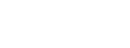Categories
Play Video

# Hamming Code Generation

After watching this video you will be able to:

No data was found

### Error correcting codes: Hamming Code

1. Hamming code is given by RW Hamming
2. It is one of the most common error correcting codes used in Random Access Memory (RAM).

### How does Hamming Code work?

1. Suppose there is a message of n bits.
2. We add k number of parity bits to the message.
3. This changes the size of the message from n bits to n+k bits.
4. The bit positions are numbered in sequence from 1 to (n+k).

for example if message length (n) is = 8, and number of parity bits (k) = 4 than total number of bits in hamming code will be (n+k) = (8+4) = 12bits

1    2    3    4    5    6    7    8    9    10    11    12

1. Those positions numbered as a power of 2 are reserved for the parity bits and the remaining bits are data bits. See below, the bits marked as red are reserved for parity bits.

1    2    3    4    5    6    7    8    9    10    11    12

### How to calculate the number of parity bits (k)?

The number of parity bits can be calculated by the following relationship

$$2^k – 1 \ge n + k$$

if the length of a message is 8 i.e. n = 8 then the value of k can be 4 or greater than 4.